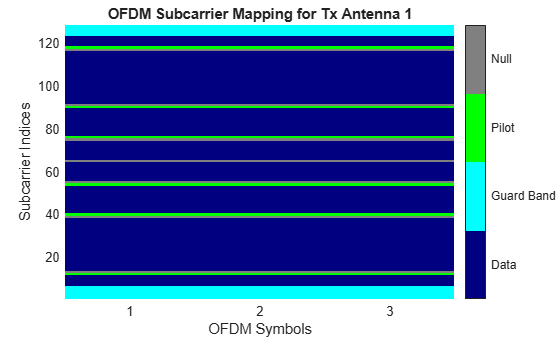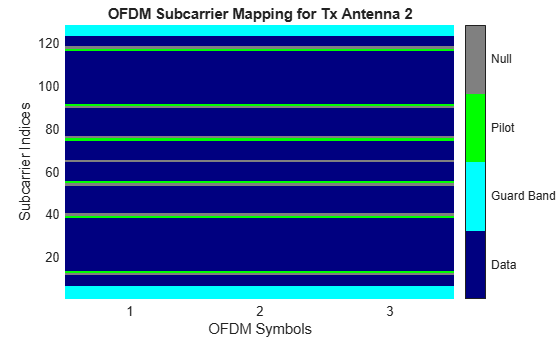# OFDM with MIMO Simulation

This example shows how to use an OFDM modulator and demodulator in a simple, 2x2 MIMO error rate simulation. The OFDM parameters are based on the 802.11n standard.

Create a QPSK modulator and demodulator pair.

```qpskMod = comm.QPSKModulator; qpskDemod = comm.QPSKDemodulator;```

Create an OFDM modulator and demodulator pair with user-specified pilot indices, an inserted DC null, two transmit antennas, and two receive antennas. Specify pilot indices that vary across antennas.

```ofdmMod = comm.OFDMModulator('FFTLength',128,'PilotInputPort',true,... 'PilotCarrierIndices',cat(3,[12; 40; 54; 76; 90; 118],... [13; 39; 55; 75; 91; 117]),'InsertDCNull',true,... 'NumTransmitAntennas',2); ofdmDemod = comm.OFDMDemodulator(ofdmMod); ofdmDemod.NumReceiveAntennas = 2;```

Show the resource mapping of pilot subcarriers for each transmit antenna. The gray lines in the figure denote the insertion of null subcarriers to minimize pilot signal interference.

`showResourceMapping(ofdmMod)`Determine the dimensions of the OFDM modulator by using the `info` method.

```ofdmModDim = info(ofdmMod); numData = ofdmModDim.DataInputSize(1); % Number of data subcarriers numSym = ofdmModDim.DataInputSize(2); % Number of OFDM symbols numTxAnt = ofdmModDim.DataInputSize(3); % Number of transmit antennas```

Generate data symbols to fill 100 OFDM frames.

```nframes = 100; data = randi([0 3],nframes*numData,numSym,numTxAnt);```

Apply QPSK modulation to the random symbols and reshape the resulting column vector to match the OFDM modulator requirements.

```modData = qpskMod(data(:)); modData = reshape(modData,nframes*numData,numSym,numTxAnt);```

Create an error rate counter.

`errorRate = comm.ErrorRate;`

Simulate the OFDM system over 100 frames assuming a flat, 2x2, Rayleigh fading channel. Remove the effects of multipath fading using a simple, least squares solution, and demodulate the OFDM waveform and QPSK data. Generate error statistics by comparing the original data with the demodulated data.

```for k = 1:nframes % Find row indices for kth OFDM frame indData = (k-1)*ofdmModDim.DataInputSize(1)+1:k*numData; % Generate random OFDM pilot symbols pilotData = complex(rand(ofdmModDim.PilotInputSize), ... rand(ofdmModDim.PilotInputSize)); % Modulate QPSK symbols using OFDM dataOFDM = ofdmMod(modData(indData,:,:),pilotData); % Create flat, i.i.d., Rayleigh fading channel chGain = complex(randn(2,2),randn(2,2))/sqrt(2); % Random 2x2 channel % Pass OFDM signal through Rayleigh and AWGN channels receivedSignal = awgn(dataOFDM*chGain,30); % Apply least squares solution to remove effects of fading channel rxSigMF = chGain.' \ receivedSignal.'; % Demodulate OFDM data receivedOFDMData = ofdmDemod(rxSigMF.'); % Demodulate QPSK data receivedData = qpskDemod(receivedOFDMData(:)); % Compute error statistics dataTmp = data(indData,:,:); errors = errorRate(dataTmp(:),receivedData); end```

Display the error statistics.

`fprintf('\nSymbol error rate = %d from %d errors in %d symbols\n',errors)`
```Symbol error rate = 9.471154e-02 from 1970 errors in 20800 symbols ```
﻿